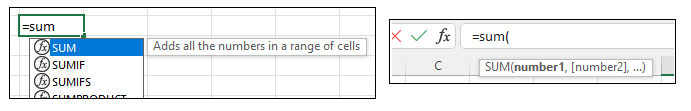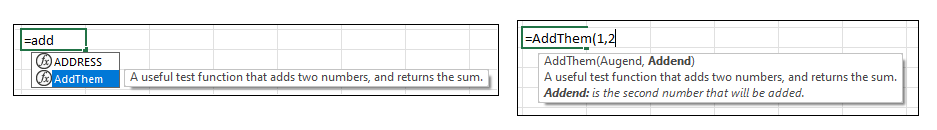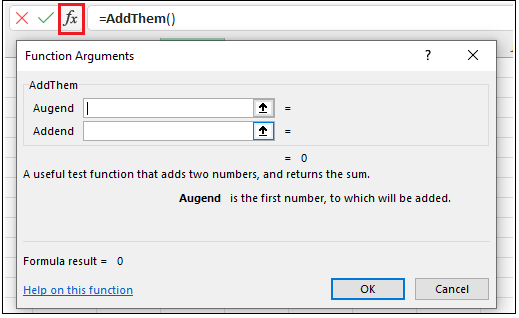# IntelliSense

Excel includes a feature that enables users to utilise functions more effectively by displaying pop-up description regarding the function and its parameters. Examples of IntelliSense in action can be seen in the figure below:By default, Excel does not provide native IntelliSense functionality to user-defined functions (UDFs). As a result, a custom Excel-DNA IntelliSense library was created as part of the Excel-DNA project.

## Usage​

Using Excel-DNA's IntelliSense capabilities is relatively simple and requires just 3 steps.

1. Depending on the language of choice, in the .csproj, .vbproj, or .fsproj file, add the following under </PropertyGroup>:
``<ItemGroup>    <PackageReference Include="ExcelDna.IntelliSense" Version="*-*" /></ItemGroup>``
1. Add a new class file to the solution by pressing Ctrl+Shift+A and include the following code:
``using ExcelDna.Integration;using ExcelDna.IntelliSense;public class IntelliSenseAddIn : IExcelAddIn{    public void AutoOpen()    {        IntelliSenseServer.Install();    }    public void AutoClose()    {        IntelliSenseServer.Uninstall();    }   }``
1. Decorate the UDF with the `ExcelFunction` and `ExcelArgument` as per the example code snippet below:
``[ExcelFunction(Description = "A useful test function that adds two numbers, and returns the sum.")]public static double AddThem(    [ExcelArgument(Name = "Augend", Description = "is the first number, to which will be added")]     double v1,    [ExcelArgument(Name = "Addend", Description = "is the second number that will be added")]         double v2){    return v1 + v2;}``

The example above would generate the following IntelliSense output:If the UDF is decorated as per step 3 above but the first 2 steps are skipped, it would still be possible to get limited information regarding the UDF. The information can be seen by writing the name of the function in Excel's Forumla Bar and clicking on the `fx` button (highlighted in red). The result can be seen in the figure below: